Get instant live expert help with Excel or Google Sheets“My Excelchat expert helped me in less than 20 minutes, saving me what would have been 5 hours of work!”

#### Post your problem and you’ll get Expert help in seconds.

Your message must be at least 40 characters
Our professional Expert are available now. Your privacy is guaranteed.

# Excel VARA Function

The VARA function in Excel estimates the variance of a sample of data, and is capable of evaluating text and logical values.  This step by step tutorial will assist all levels of Excel users in the usage and syntax of the VARA function.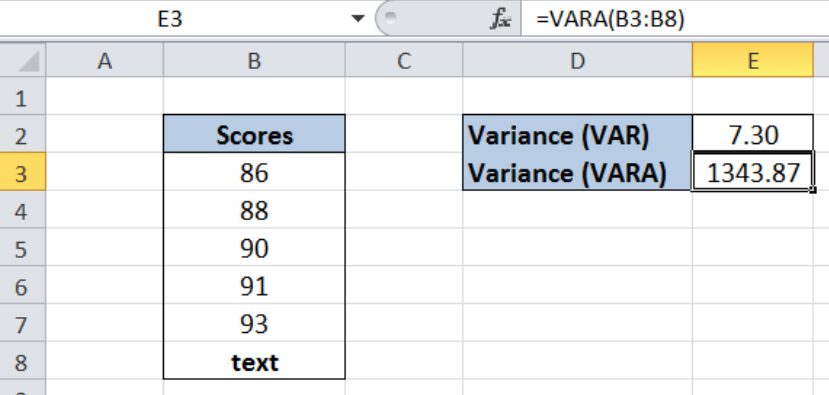Figure 1. Final result: Excel VARA function

Final formula: `=VARA(B3:B8)`

Syntax of the VARA Function

`=VARA(number1,[number2],...])`

• number1 – any number, array or cell reference representing a sample of a population whose variance we want to calculate
• Only number1 is required; succeeding numbers are optional

## Setting up the Data

We have here a list of Scores in column B.  We leave cell B8 empty for now.  In cell E2, the variance of the scores is calculated using the VAR function, which is 7.30.  In cell E3, we want to calculate the variance by using the VARA function, and differentiate the two functions:  VAR and VARA.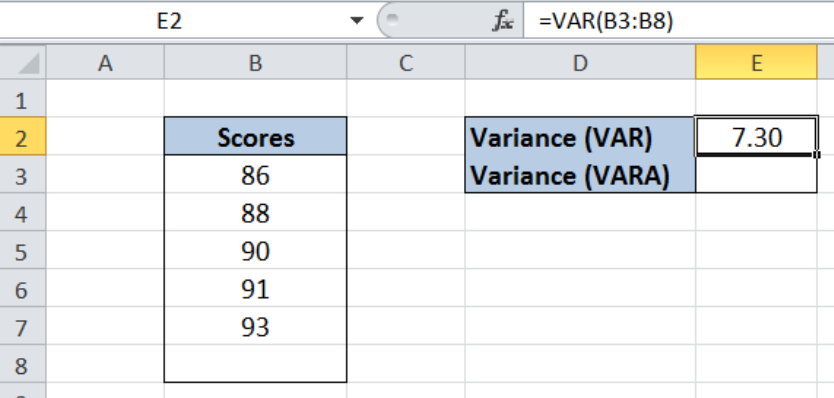Figure 2. Sample data to calculate variance using VARA function

## Calculate variance using VARA function

In this example, we will learn how to calculate variance using the VARA function, and show the difference between VARA and VAR functions.  Let us follow these steps:

Step 1.  Enter the text string “text” in cell B8.

Step 2.  Select cell E3

Step 3.  Enter the formula: `=VARA(B3:B8)`

Step 4. Press Enter

The range B3:B8 contains our data.  Note that the VAR formula ignores the text we have entered in cell B8, and returns the same value for variance.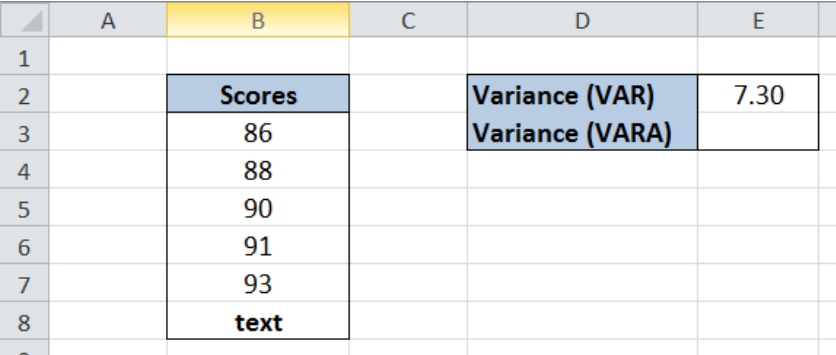Figure 3. VAR function ignores the text value in cell B8

On the other hand, the VARA function evaluates the text as a zero “0” and calculates the variance.

As a result, the variance in cell E3 is 1343.87.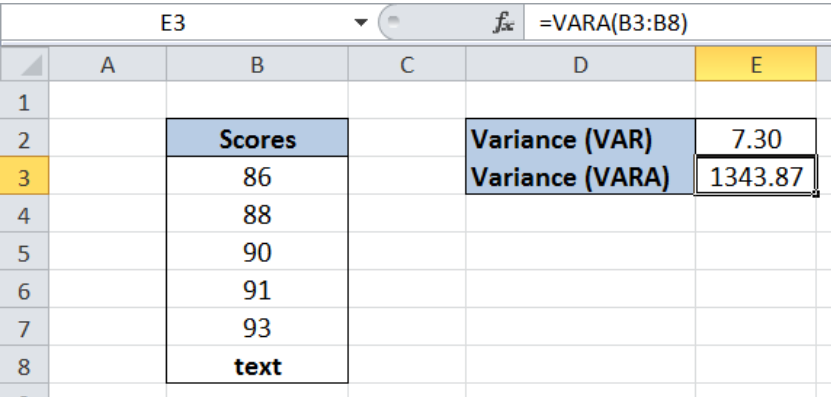Figure 4. Output: Calculate variance using VARA function

## Notes:

• VARA function evaluates logical values {TRUE or FALSE} and text values
• Arguments containing TRUE are evaluated as 1, while those that contain text values or the logical value FALSE are evaluated as zero “0”
• We use VARA or VAR when we have a sample of the data; if our data represents the entire population, we use VARP
• Below table shows the comparison of VAR, VARA and VARP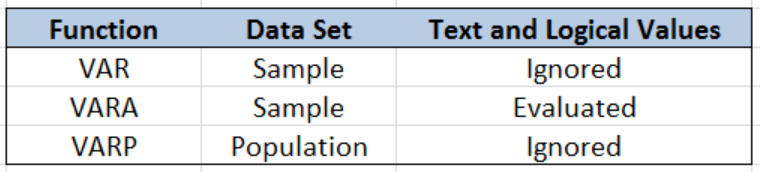Figure 5. Comparison: VAR, VARA and VARP

Most of the time, the problem you will need to solve will be more complex than a simple application of a formula or function. If you want to save hours of research and frustration, try our live Excelchat service! Our Excel Experts are available 24/7 to answer any Excel question you may have. We guarantee a connection within 30 seconds and a customized solution within 20 minutes.

Solution examplesUse the Vlookup Function to complete the "employee" column of table 2. Use "job Id" from table 2 as your lookup_value(s) and table 1 as your reference.
Solved by C. H. in 16 minsIf a cell in another sheet is populated I need a vlookup done. If the cell is not populated I need the cell to return blank.
Solved by T. D. in 60 minsI am trying to make a chart that turns a week range red if nothing is entered in the range. If something is entered then I would like it to turn green. Please Help
Solved by E. U. in 43 minsI need a check box to show/hide an answer of an if function
Solved by Z. U. in 23 minsI need a formula to compare the data in two columns and then export the mismatched data in the 3rd column
Solved by S. Q. in 20 mins